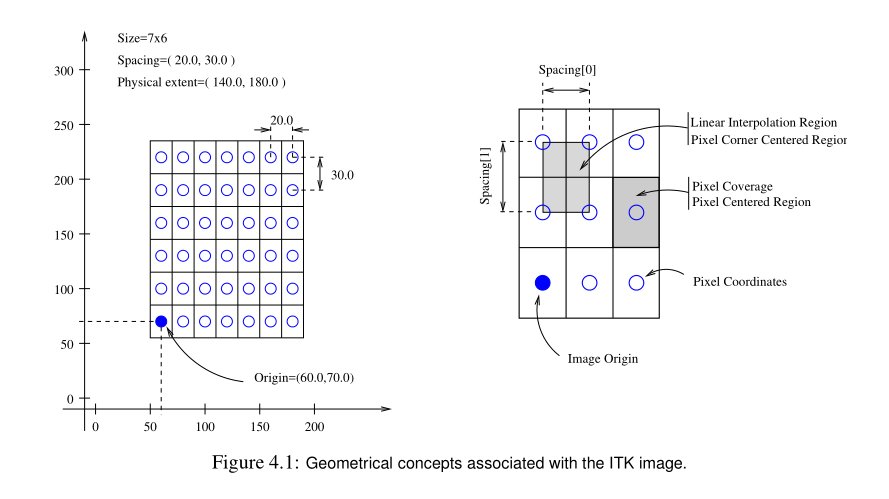## ITK 定义原点和间距 Defining Origin and Spacing

2020/9/19 15:44:05 文章标签:#include "ItkImage.h"

static itk::Image<unsigned short, 3>::IndexType GetIndexFromMouseClick()
{
itk::Image<unsigned short, 3>::IndexType LeftEyeIndex;
LeftEyeIndex = 60;
LeftEyeIndex = 127;
LeftEyeIndex = 93;
return LeftEyeIndex;
}

int main(int, char* [])
{
constexpr unsigned int Dimension = 3;
using ImageType = itk::Image<unsigned short, Dimension>;

ImageType::Pointer image = ImageType::New();

//初始化图片区域
const ImageType::SizeType size = { {200,200,200} };
const ImageType::IndexType start = { {0,0,0} };

/*以上两句话可以写成如下来初始化图片区域
ImageType::SizeType size;
size = 200;
size = 200;
size = 200;

ImageType::IndexType start;
start = 0;
start = 0;
start = 0;
*/

//定义起始索引和图像大小之后，这两个参数用于创建一个itk::ImageRegion对象
//该对象基本上封装了这两个概念
//该区域由初始索引和图像大小初始化
ImageType::RegionType region;
region.SetSize(size);
region.SetIndex(start);

//像素数据分配
image->SetRegions(region);
//将缓冲区初始化为零
image->Allocate(true);

//图像间距用一个固定数组表示，其大小与图像的维数相匹配
ImageType::SpacingType spacing;

//单位(例如，mm，英寸等)是由应用程序定义的
spacing = 0.33; // spacing along X
spacing = 0.33; // spacing along Y
spacing = 1.20; // spacing along Z

//可以使用SetSpacing()方法将数组分配给图像
image->SetSpacing(spacing);

//使用GetSpacing()方法从图像检索间距信息
//此方法返回对FixedArray的引用
//然后可以使用返回的对象读取数组的内容
//注意，使用const关键字表示不会修改数组
const ImageType::SpacingType& sp = image->GetSpacing();

std::cout << "Spacing=";
std::cout << sp << "," << sp << "," << sp << std::endl;

//下面的代码演示了如何创建和分配适合初始化映像源的变量
//第一个像素的中心在N-D的坐标
ImageType::PointType newOrigin;
newOrigin.Fill(0.0);
image->SetOrigin(newOrigin);

//还可以使用GetOrigin()方法从图像中检索原点
// 这将返回对一个点的引用
//该引用可用于读取数组的内容
//再次注意，使用const关键字表示不会修改数组内容
const ImageType::PointType& origin = image->GetOrigin();
std::cout << "Origin = ";
std::cout << origin << ", " << origin << ", " << origin << std::endl;

//下面的代码演示了如何创建和分配适合用标识初始化图像方向的变量
//第一个像素的中心在N-D的坐标
ImageType::DirectionType direction;
direction.SetIdentity();
image->SetDirection(direction);

//还可以使用GetDirection()方法从图像中检索方向
//这将返回一个对矩阵的引用
//该引用可用于读取数组的内容
//再次注意，使用const关键字表示不能修改矩阵内容
const  ImageType::DirectionType & direct = image->GetDirection();

std::cout << "Direction=" << std::endl;
std::cout << direct << std::endl;

//一旦图像样本的间距、原点和方向被初始化，图像将正确地从物理空间坐标映射像素索引
//下面的代码说明了如何将物理空间中的点映射到图像索引中，以便读取最近像素的内容
//首先，必须声明itk::Point类型
//点类型被模板化在用于表示坐标的类型和空间的维度上
//在这种特殊情况下，点的维数必须与图像的维数匹配
using PointType = itk::Point<double, ImageType::ImageDimension>;
PointType point;
point = 1.45; // x 坐标
point = 7.21; // y 坐标
point = 9.28; // z 坐标

//须提供一个索引对象来接收映射的结果
//可以使用在图像类型中定义的IndexType实例化索引对象
ImageType::IndexType pixelIndex;

//image类的TransformPhysicalPointToIndex()方法将计算最接近所提供点的像素索引
//该方法检查该索引是否包含在当前缓冲的像素数据中
//该方法返回一个布尔值，指示结果索引是否位于缓冲区域内
//当方法的返回值为false时，不应使用输出索引

const bool isInside = image->TransformPhysicalPointToIndex(point, pixelIndex);

//如果TransformPhysicalPointToIndex（）方法返回True时
if (isInside)
{
//GetPixel()和SetPixel()是访问像素数据的效率非常低的方法
ImageType::PixelType pixelValue;
pixelValue = image->GetPixel(pixelIndex);
pixelValue += 5;
image->SetPixel(pixelIndex, pixelValue);
}

//GetIndexFromMouseClick()为自定义方法，终端用户使用鼠标界面手动选择体素索引位置
const ImageType::IndexType LeftEyeIndex = GetIndexFromMouseClick();
ImageType::PointType LeftEyePoint;
//TransformIndexToPhysicalPoint（）方法：
//从一个离散索引(在索引空间中)获得一个物理点(在原点和间隔信息来自的空间中)
image->TransformIndexToPhysicalPoint(LeftEyeIndex, LeftEyePoint);

std::cout << "===========================================" << std::endl;
std::cout << "The Left Eye Location is " << LeftEyePoint << std::endl;

/*这是一个矩阵
P1          O1        D1,1  D1,2  D1,3           S1 0 0           I1
P2    =     O2    +   D2,1  D2,2  D2,3      ∗    0 S2 0      ∗    I2
P3          O3        D3,1  D3,2  D3,3           0 0 S3           I3
*/
//一个Dimendion*Dimension的double类型的矩阵
using MatrixType = itk::Matrix<double, Dimension, Dimension>;
MatrixType SpacingMatrix;
//矩阵中填充数值
SpacingMatrix.Fill(0.0F);
//得到数据放到ImageSpacing数组中
const ImageType::SpacingType& ImageSpacing = image->GetSpacing();

SpacingMatrix(0, 0) = ImageSpacing;
SpacingMatrix(1, 1) = ImageSpacing;
SpacingMatrix(2, 2) = ImageSpacing;

const ImageType::DirectionType& ImageDirectionCosines = image->GetDirection();
const ImageType::PointType& ImageOrigin = image->GetOrigin();

//这段代码我也有点不太明白
using VectorType = itk::Vector<double, Dimension>;
VectorType LeftEyeIndexVector;
LeftEyeIndexVector = LeftEyeIndex;
LeftEyeIndexVector = LeftEyeIndex;
LeftEyeIndexVector = LeftEyeIndex;
ImageType::PointType LeftEyePointByHand = ImageOrigin + ImageDirectionCosines * SpacingMatrix * LeftEyeIndexVector;

return EXIT_SUCCESS;
}

暂无相关的数据...